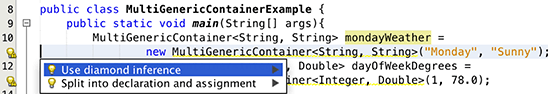# 泛型：工作原理及其重要性

2014 年 7 月发布

Java SE 8 的发布曾在 Java 界引起轰动。该版本中新增的和更新的语言特性可减少需要编写的代码量并使代码更易于使用，从而提高开发人员的工作效率。要充分了解一些新特性（如 lambda）的实现，您需要先了解该语言的核心概念。其中一个在许多 Java SE 8 特性中发挥了重要作用的概念是泛型。

GitHub 上提供了本文的完整源代码。

## 泛型是什么？

```public class ObjectContainer {
private Object obj;

/**
* @return the obj
*/
public Object getObj() {
return obj;
}

/**
* @param obj the obj to set
*/
public void setObj(Object obj) {
this.obj = obj;
}

}
```

```ObjectContainer myObj = new ObjectContainer();

// store a string
myObj.setObj("Test");
System.out.println("Value of myObj:" + myObj.getObj());
// store an int (which is autoboxed to an Integer object)
myObj.setObj(3);
System.out.println("Value of myObj:" + myObj.getObj());

List objectList = new ArrayList();
// We have to cast and must cast the correct type to avoid ClassCastException!
String myStr = (String) ((ObjectContainer)objectList.get(0)).getObj();
System.out.println("myStr: " + myStr);
```

```public class GenericContainer<T> {
private T obj;

public GenericContainer(){
}

// Pass type in as parameter to constructor
public GenericContainer(T t){
obj = t;
}

/**
* @return the obj
*/
public T getObj() {
return obj;
}

/**
* @param obj the obj to set
*/
public void setObj(T t) {
obj = t;
}
}
```

```GenericContainer<Integer> myInt =  new GenericContainer<Integer>();
```

```myInt.setObj(3);  // OK
myInt.setObj("Int"); // Won't Compile
```

## 使用泛型的好处

```List myObjList = new ArrayList();

// Store instances of ObjectContainer
for(int x=0; x <=10; x++){
ObjectContainer myObj = new ObjectContainer();
myObj.setObj("Test" + x);
}
// Get the objects we need to cast
for(int x=0; x <= myObjList.size()-1; x++){
ObjectContainer obj = (ObjectContainer) myObjList.get(x);
System.out.println("Object Value: " + obj.getObj());
}

List<GenericContainer> genericList = new ArrayList<GenericContainer>();

// Store instances of GenericContainer
for(int x=0; x <=10; x++){
GenericContainer<String> myGeneric = new GenericContainer<String>();
myGeneric.setObj(" Generic Test" + x);
}
// Get the objects; no need to cast to String

for(GenericContainer<String> obj:genericList){
String objectString = obj.getObj();
// Do something with the string...here we will print it
System.out.println(objectString);
}
```

## 分析泛型

### 如何使用泛型？

```public class GenericContainer<T> {
...
```

• `E`：元素
• `K`：键
• `N`：数字
• `T`：类型
• `V`：值
• `S``U``V` 等：多参数情况中的第 2、3、4 个类型

```GenericContainer<String> stringContainer = new GenericContainer<String>();
```

```GenericContainer gc1 = new GenericContainer(3);
GenericContainer gc2 = new GenericContainer("Hello");
```

```GenericContainer rawContainer = new GenericContainer();
```

### 多种泛型类型

```public class MultiGenericContainer<T, S> {
private T firstPosition;
private S secondPosition;

public MultiGenericContainer(T firstPosition, S secondPosition){
this.firstPosition = firstPosition;
this.secondPosition = secondPosition;
}

public T getFirstPosition(){
return firstPosition;
}

public void setFirstPosition(T firstPosition){
this.firstPosition = firstPosition;
}

public S getSecondPosition(){
return secondPosition;
}

public void setSecondPosition(S secondPosition){
this.secondPosition = secondPosition;
}

}
```

`MultiGenericContainer` 类可用于存储两个不同对象，每个对象的类型可在实例化时指定。容器的用法如清单 6 所示。

```MultiGenericContainer<String, String> mondayWeather =
new MultiGenericContainer<String, String>("Monday", "Sunny");
MultiGenericContainer<Integer, Double> dayOfWeekDegrees =
new MultiGenericContainer<Integer, Double>(1, 78.0);

String mondayForecast = mondayWeather.getFirstPosition();
// The Double type is unboxed--to double, in this case. More on this in next section!
double sundayDegrees = dayOfWeekDegrees.getSecondPosition();
```

### 类型推断和尖括号运算符

```MultiGenericContainer<String, String> mondayWeather =
new MultiGenericContainer<String, String>("Monday", "Sunny");
MultiGenericContainer<Integer, Double> dayOfWeekDegrees =
new MultiGenericContainer<Integer, Double>(1, 78.0);
String mondayForecast = mondayWeather.getFirstPosition(); // Works fine with String
// The following generates "Incompatible types" error and won't compile
int mondayOutlook = mondayWeather.getSecondPosition();
double sundayDegrees = dayOfWeekDegrees.getSecondPosition(); // Unboxing occurs
```

：无法将原始类型用于泛型；只能使用引用类型。自动装箱和拆箱操作能够在使用泛型对象时将值存储为原始类型并检索原始类型的值。

```MultiGenericContainer<String, String> mondayWeather =
new MultiGenericContainer<>("Monday", "Sunny");
MultiGenericContainer<Integer, Double> dayOfWeekDegrees =
new MultiGenericContainer<>(1, 78.0);
```### 我的目标是什么？

```double sundayDegrees = dayOfWeekDegrees.getSecondPosition();
```

### 有界类型

```<T extends UpperBoundType>
```

```<T super LowerBoundType>
```

```public class GenericNumberContainer <T extends Number> {
private T obj;

public GenericNumberContainer(){
}

public GenericNumberContainer(T t){
obj = t;
}
/**
* @return
the obj
*/
public T getObj() {
return obj;
}

/**
* @param obj the obj to set
*/
public void setObj(T t) {
obj = t;
}
}
```

```GenericNumberContainer<Integer> gn = new GenericNumberContainer<Integer>();
gn.setObj(3);

// Type argument String is not within the upper bounds of type variable T
GenericNumberContainer<String> gn2 = new GenericNumberContainer<String>();
```

### 泛型方法

```public static <N extends Number> double add(N a, N b){
double sum = 0;
sum = a.doubleValue() + b.doubleValue();
return sum;
}
```

```double genericValue1 = Calculator.add(3, 3f);
```

### 通配符

```public static <T> void checkList(List<?> myList, T obj){
if(myList.contains(obj)){
System.out.println("The list contains the element: " + obj);
} else {
System.out.println("The list does not contain the element: " + obj);
}
}
```

```// Create List of type Integer
List<Integer> intList = new ArrayList<Integer>();

// Create List of type String
List<String> strList = new ArrayList<String>();

// Create List of type Object
List<Object> objList = new ArrayList<Object>();

checkList(intList, 3);
// Output:  The list [2, 4, 6] does not contain the element: 3

checkList(objList, strList);
/* Output:  The list [two, four, [two, four, six]] contains
the element: [two, four, six] */

checkList(strList, objList);
/* Output:  The list [two, four, six] does not contain
the element: [two, four, [two, four, six]] */
```

```public static <T> void checkNumber(List<? extends Number> myList, T obj){
if(myList.contains(obj)){
System.out.println("The list " + myList + " contains the element: " + obj);
} else {
System.out.println("The list " + myList + " does not contain the
element: " + obj);
}
}
```

## 在 Java SE 8 构造中使用泛型

```@FunctionalInterface
public interface Predicate<T>{
...
}
```

```public static void compareStrings(List<String> list, Predicate<String> predicate) {
list.stream().filter((n) -> (predicate.test(n))).forEach((n) -> {
System.out.println(n + " ");
});
}
```

```List<String> bookList = new ArrayList<>();
compareStrings(bookList, (n)->n.contains("Java EE"));
```

## 更进一步

```public <T> long countTypes(T coffeeType) {
long count = purchase.stream().filter(
(sale) -> (sale.getType().getType().equals(coffeeType)))
.count();
return count;
}
```

```public <T extends CoffeeSaleType> void addToPurchase(List<T> saleList) {
for (CoffeeSaleType sale : saleList) {
}
}
```

## 关于作者

Josh Juneau 担任应用开发人员、系统分析师和数据库管理员。他主要使用 Java 和其他 Java 虚拟机 (JVM) 语言开发。他是 Oracle 技术网和 Java Magazine 的技术作家，与人合著了《The Definitive Guide to Jython》和《PL/SQL Recipes》（均为 Apress，2010）和《Java 7 Recipes》（Apress，2011）。Josh 最近撰写了《Java EE 7 Recipes》和《Introducing Java EE 7》（均为 Apress，2013），他目前正在写一本 Apress 的书《Java 8 Recipes》，将于今年晚些时候出版。